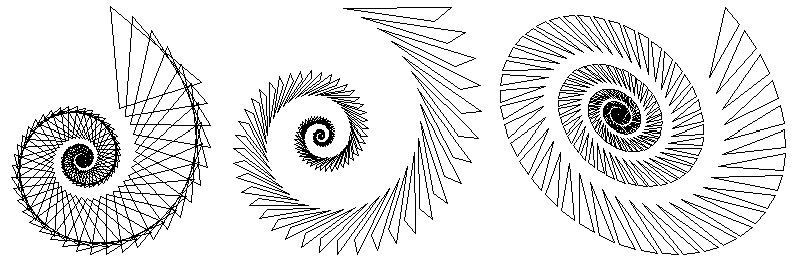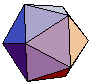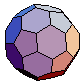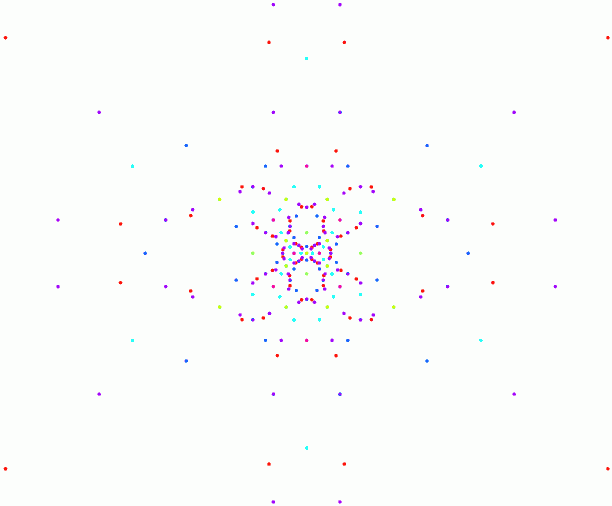Math Games Matrix Revolutions Ed Pegg Jr., November 10, 2003

In bullet-time, a careful arrangement of cameras gives the illusion of movement as the world stays still. Computer games allow joysticks and controllers to change a point of view in response to player actions. In computer-generated movies, the characters and their homes all move around in a believable way.

Movement, in these cases, relies on matrices.

A matrix, very simply, is a rectangular array of numbers. Joseph James Sylvester developed matrices in 1851, and described many of their properties. As linear algebra was further developed, Charles Lutwidge Dodgson (AKA Lewis Carroll) objected to the term "matrix." He preferred the term "block," and argued this point in his book Elementary Treatise on Determinants -- his first book after Alice in Wonderland. Ironically, the fictional character Neo is told to “follow the white rabbit” in a movie that was not called "The Block".

Here are some sample matrices.Figure 1. Sample matrices.

Here are pictures illustrating what these matrices can do.Figure 2. Rotation, reflection, and translation via matrices.

In image 2A, the point p can be seen at {2,3}. In the image 2B, p undergoes a rotation to a new point p', via the operation p' = B p. The matrix B is a rotation matrix, which rotates the given point p in an arc centered on the origin, {0,0}. Image 2C gives a reflection of the point p, which can be calculated by p' = C p. So far, all of of these actions have involved matrix multiplication. Image 2D shows an example of translation, which can be represented by p' = q + p, a case of matrix addition. Here's a quick reminder of how matrix multiplication works.Figure 3. Matrix multiplication.

Objects can be broken up into points. For example, it is now possible to print 3D sculptures in steel and bronze, point by point. With three points, a triangle can be made, and the concept of scaling can be introduced. For many computer animations, that is enough. Rotation, Reflection, Translation, and Scaling are the main tools of making nice graphics with matrices. As an example, I took some random triangles, and multiplied them by rotation and scaling matrices hundreds of times. trin+1 = S R tri n, with n going to 300. S is a scaling matrix. R is a rotation matrix. In the third image, a translation matrix was added at each step, which resulted in a more elliptical spiral. As a side note, I didn't know what would happen in the last case. I just tried it.Figure 4. Effects of rotation, scaling, and translation on random triangles.

It's all very simple -- just let the computer perform a few hundred matrix computations. This process works in 3 dimensions, as well. At the Hyperstar site, you can see slices of 4D space. Back in 3D space, here is a simple shell skeleton, made with 6 random points, a 3D rotation matrix, and a .99 scaling matrix.Figure 5. 3D rotation and scaling on 6 random points.

Without scaling, a set of rotation matrices can pick out points on a circle (in 2D) or a sphere (in 3D). For example, using rotation matrix B a thousand times only produces six points. B raised to powers 0 through 6 are listed in figure 6 -- if any two of these matrices are multiplied together, one of these six matrices will be the result. As such, these 6 matrices form what is called a group. This group is named the order 6 cyclic group, or C6.Figure 6. A group of rotation matrices.

Groups allow mathematicians to study symmetry of all types. C6 is an example of a finite group. All the 2D rotation matrices containing only rational numbers and radicals combine to make an infinite group -- the angles constructible with a straightedge and compass. Curiously, this infinite group does not contain the matrix corresponding to a 20 degree rotation. There are many different infinite groups.

The set of all 3D rotation matrices is named the special orthogonal group, or SO(3). If you have a sphere handy, this group represents all the ways the sphere can be turned without changing the center point. This is another infinite group. For finite subgroups, the C6 group above is an example. If the right reflection matrix is added, the dihedral group D6 can be made. There are three more, the tetrahedral group T, the octahedral group O, and the icosahedral group I. These groups correspond to the platonic solids. For instance, the cube has the same properties as O.

Of these three, I is the most complicated. Let φ be the Golden Ratio, (Sqrt+1)/2. The twelve vertices of an icosahedron can be represented as {{0,φ,1}, {0,φ,-1}, {0,-φ,1}, {0,-φ,-1}, {1,0,φ}, {-1,0,φ}, {1,0,-φ}, {-1,0,-φ}, {φ,1,0}, {φ,-1,0}, {-φ,1,0}, {-φ,-1,0}}. Using these twelve points, the twenty triangles are {{1,2,9}, {1,2,11}, {1,5,9}, {1,5,6}, {1,6,11}, {2,7,9}, {2,8,11}, {5,9,10}, {3,5,6}, {6,11,12}, {7,9,10}, {2,7,8}, {8,11,12}, {3,5,10}, {3,6,12}, {4,7,10}, {4,7,8}, {4,8,12}, {3,4,10}, {3,4,12}}. Each triangle has three rotations. Pick one, and find all the rotation matrices that maps this initial triangle to each of the 60 possibilities. That set of 60 matrices is I, the icosahedral group. No matter which two matrices are chosen, their matrix product will be a member of I.

With I, it is easy to describe various Archimedean solids. For example,the vertices of the truncated dodecahedron are found with I • {1,2,2-φ}. Groups are very useful! The figure below gives all the Archimedean solids realizable with I. The snub dodecahedron was rather tricky to solve -- a is a root of x6 + 6x5 - 7x4 - 9x3 - 14x2 - 7x -1, and b is a root of x6 - 2x5 - x4 + x3 + 2x2 + x -1. There are 60 solutions for the snub in the form {1, a, b}.{1,0,φ} {1,1,1} {1,0,0} {1,0,3φ} {1,2,2-φ} {1,2,φ} {1,a,b} icosahedron dodecahedron icosidodecahedron truncated icosahedron truncated dodecahedron small rhombicosidodecahedron snub dodecahedron

Figure 7. How a single vertex and I can make an archimedean solid.

If a reflection matrix is added, the group order becomes 120. Vertices of the Great Rhombicosidodecahedron can be found with that group and {1,1,4φ+1}. Have you noticed that all of the vertices I've given so far of of the form {1,x,y}? Figure 8 charts out all the {x,y} pairs that give an archimedean solid.Figure 8. The {x,y} points corresponding to an Archimedean solid representable as I • {1,x,y}.

All in all, matrix revolutions are very handy. To see a java implementation tying all this together, try out Jim Morey's Archimedean Kaleidoscope.

References:

Lomont, J. S. Applications of Finite Groups. Dover Publications, Inc. 1987.

MacTutor History of Mathematics. James Joseph Sylvester, Charles Lutwidge Dodgson, http://turnbull.mcs.st-and.ac.uk/history/.

Weisstein, Eric W. Rotation Matrix, Group, Matrix, Icosahedral Group. Eric Weisstein's World of Mathematics. http://mathworld.wolfram.com/.

Yale. P. B. Geometry and Symmetry. Dover Publications, Inc. 1968.

Mathematica Code:

A notebook for this column is available at the Mathematica Information Center.

<< Geometry`Rotations` (*Preload Rotations package*)

(*Figure 4: *) Show[Graphics[Map[Line, NestList[Map[{{.97, 0}, {0, .97}}.RotationMatrix2D[N[Pi/20]].# &, #] &, With[{k = Table[Random[], {3}, {2}]}, Append[k, First[k]]], 300]], AspectRatio -> Automatic, PlotRange -> {All, All}]];

(*Figure 5: *) Show[Graphics3D[With[{gg = NestList[Map[{{.99, 0, 0}, {0, .99, 0}, {0, 0, .99}}.RotationMatrix3D[Pi/31, Pi/53, Pi/47].# &, #] &, With[{k = Table[Random[Real, {-3, -4}], {6}, {3}]}, Append[k, First[k]]], 500]}, {Map[Line, gg], Map[Line, Transpose[gg]]}], AspectRatio -> Automatic, PlotRange -> {All, All, All}, Boxed -> False]];

(*Figure 7 and 8: See http://library.wolfram.com/infocenter/MathSource/4807/*)

Comments are welcome. Please send comments to Ed Pegg Jr. at ed@mathpuzzle.com.

Ed Pegg Jr. is the webmaster for mathpuzzle.com. He works at Wolfram Research, Inc. as the administrator of the Mathematica Information Center.